# Random Number Generators 1 Random Number Generators Random

• Slides: 32Random Number Generators 1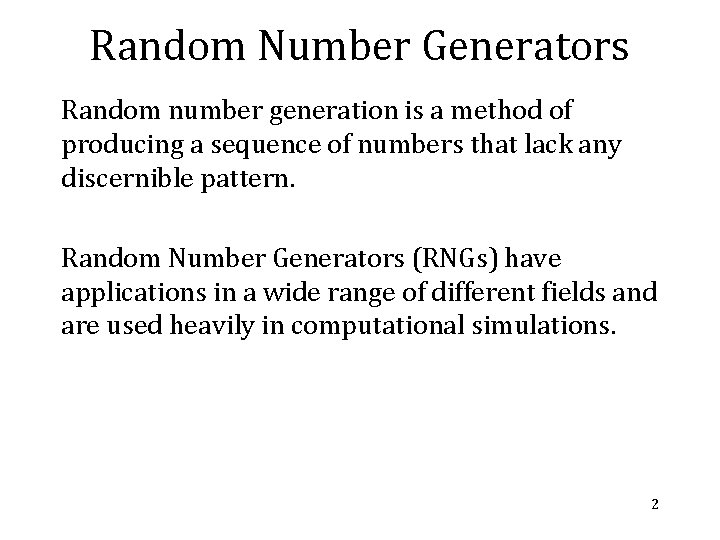Random Number Generators Random number generation is a method of producing a sequence of numbers that lack any discernible pattern. Random Number Generators (RNGs) have applications in a wide range of different fields and are used heavily in computational simulations. 2What is Random? What do we mean by Random? • Uncorrelated (with what you're interested in) • Quantum systems are truly random (we believe) • Some times we want repeatability from a random number generator. 3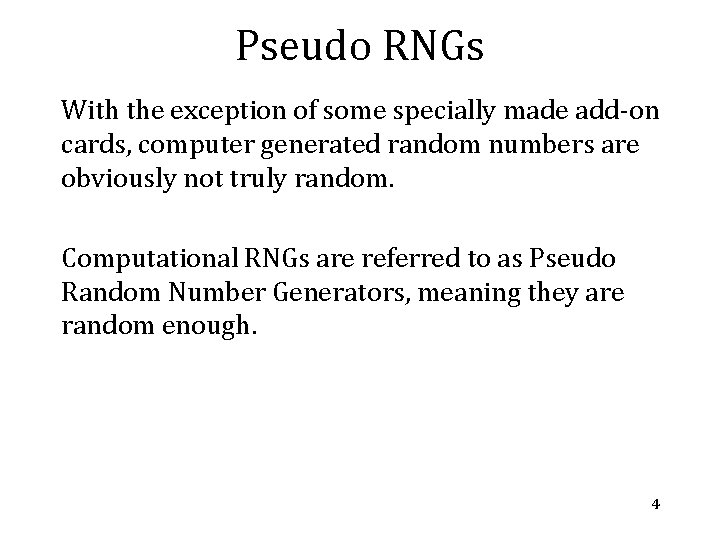Pseudo RNGs With the exception of some specially made add-on cards, computer generated random numbers are obviously not truly random. Computational RNGs are referred to as Pseudo Random Number Generators, meaning they are random enough. 4Pseudo RNGs PRNGs usually generate very long sequences of random numbers. For example the commonly used Mersenne Twister MT 19937 has a sequence length of 219937 -1. Basically a sequence that will not recur in any practice compute time – eg the lifetime of the known universe. 5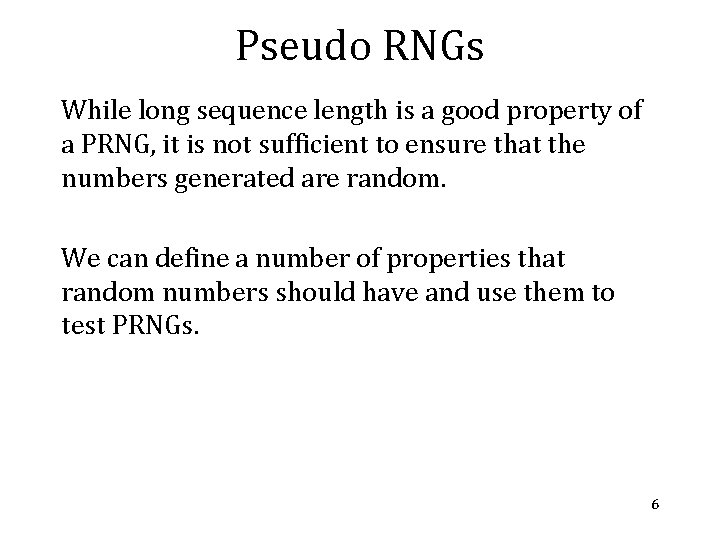Pseudo RNGs While long sequence length is a good property of a PRNG, it is not sufficient to ensure that the numbers generated are random. We can define a number of properties that random numbers should have and use them to test PRNGs. 6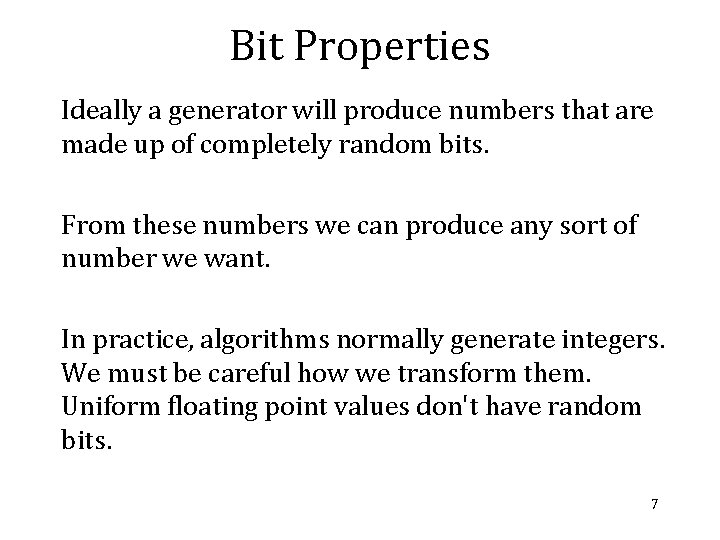Bit Properties Ideally a generator will produce numbers that are made up of completely random bits. From these numbers we can produce any sort of number we want. In practice, algorithms normally generate integers. We must be careful how we transform them. Uniform floating point values don't have random bits. 7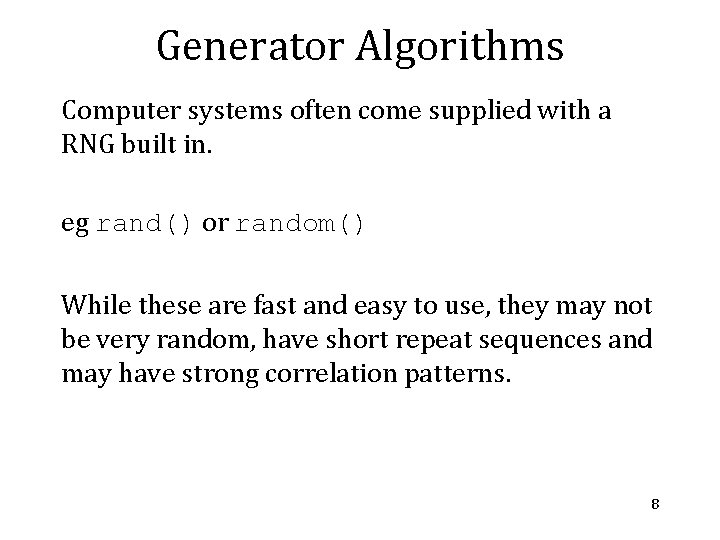Generator Algorithms Computer systems often come supplied with a RNG built in. eg rand() or random() While these are fast and easy to use, they may not be very random, have short repeat sequences and may have strong correlation patterns. 8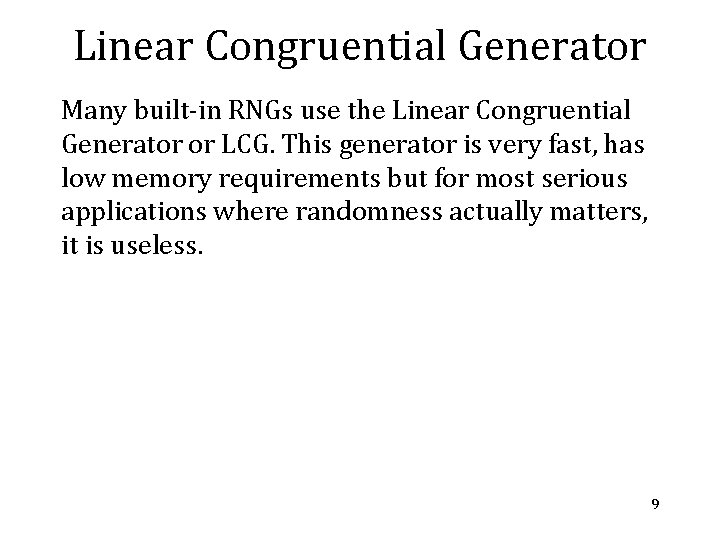Linear Congruential Generator Many built-in RNGs use the Linear Congruential Generator or LCG. This generator is very fast, has low memory requirements but for most serious applications where randomness actually matters, it is useless. 9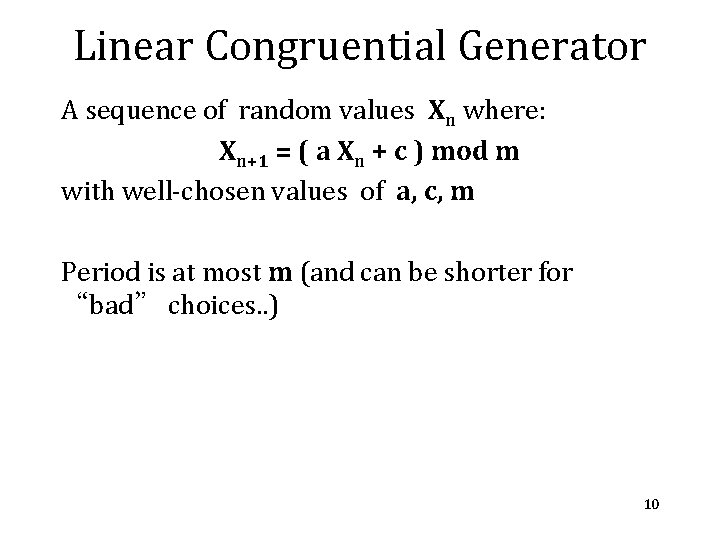Linear Congruential Generator A sequence of random values Xn where: Xn+1 = ( a Xn + c ) mod m with well-chosen values of a, c, m Period is at most m (and can be shorter for “bad” choices. . ) 10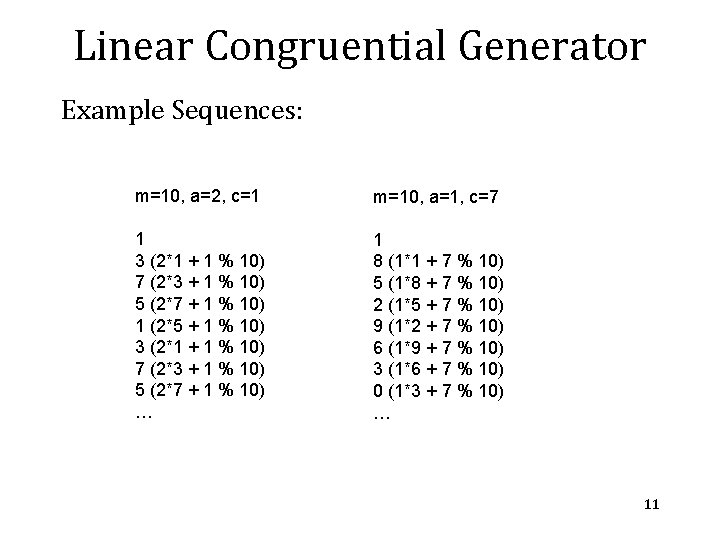Linear Congruential Generator Example Sequences: m=10, a=2, c=1 m=10, a=1, c=7 1 3 (2*1 + 1 % 10) 7 (2*3 + 1 % 10) 5 (2*7 + 1 % 10) 1 (2*5 + 1 % 10) 3 (2*1 + 1 % 10) 7 (2*3 + 1 % 10) 5 (2*7 + 1 % 10) … 1 8 (1*1 + 7 % 10) 5 (1*8 + 7 % 10) 2 (1*5 + 7 % 10) 9 (1*2 + 7 % 10) 6 (1*9 + 7 % 10) 3 (1*6 + 7 % 10) 0 (1*3 + 7 % 10) … 11Linear Congruential Generator Various sources use different parameters for the LCG: Numerical Recipes m = 232 a = 1664525 c = 1013904223 GCC m = 232 a = 1103515245 c = 12345 MMIX m = 264 a = 6364136223846793005 c = 1442695040888963407 12Lagged Fibonacci Generator The Lagged Fibonacci Generator is based on a generalisation of the Fibonacci sequence 1, 1, 2, 3, 5, 8, 13, 21. . Obtained from the sequence Xn = Xn-1 + Xn-2 13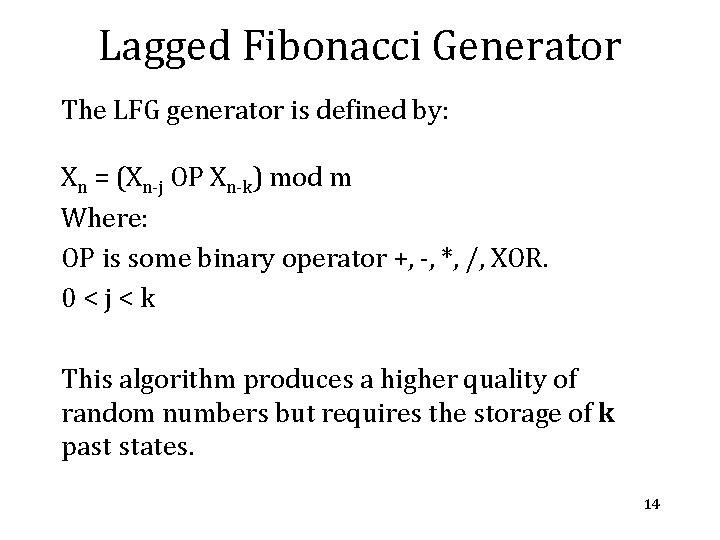Lagged Fibonacci Generator The LFG generator is defined by: Xn = (Xn-j OP Xn-k) mod m Where: OP is some binary operator +, -, *, /, XOR. 0<j<k This algorithm produces a higher quality of random numbers but requires the storage of k past states. 14Mersenne Twister The Mersenne Twister is a widely used PRNG, the name comes from the period length which is chosen to be a Mersenne Prime. The commonly used MT 19937 algorithm stores and generates 624 at a time and can extract them one by one. See: http: //www. math. sci. hiroshima-u. ac. jp/~m-mat/MT/emt. html 15Distributions PRNGs usually produce random unsigned integers which can easily be converted to a uniform distribution [0, 1). What about other distributions? Gaussian or Normal distribution is common. (Box-Muller transformation can transform a pair of uniform deviates into a Gaussian pair). Poissonian? Binomial? 16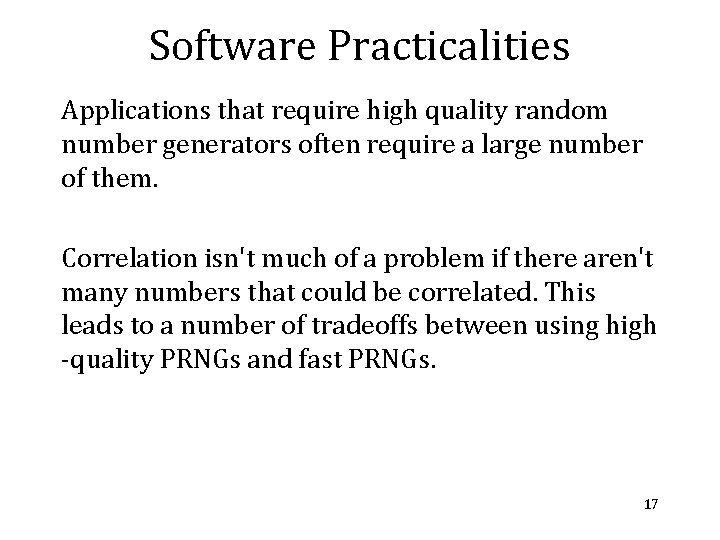Software Practicalities Applications that require high quality random number generators often require a large number of them. Correlation isn't much of a problem if there aren't many numbers that could be correlated. This leads to a number of tradeoffs between using high -quality PRNGs and fast PRNGs. 17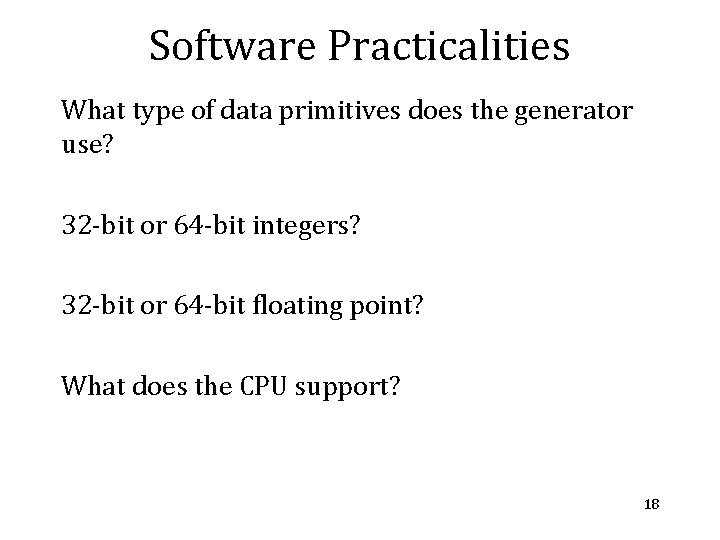Software Practicalities What type of data primitives does the generator use? 32 -bit or 64 -bit integers? 32 -bit or 64 -bit floating point? What does the CPU support? 18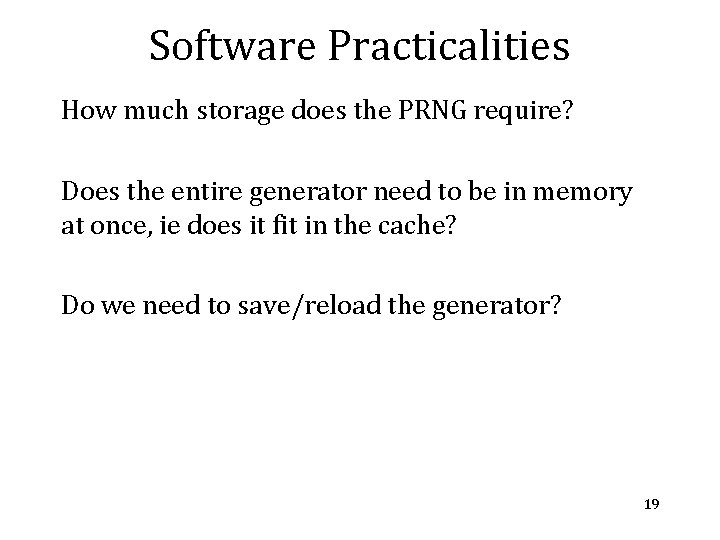Software Practicalities How much storage does the PRNG require? Does the entire generator need to be in memory at once, ie does it fit in the cache? Do we need to save/reload the generator? 19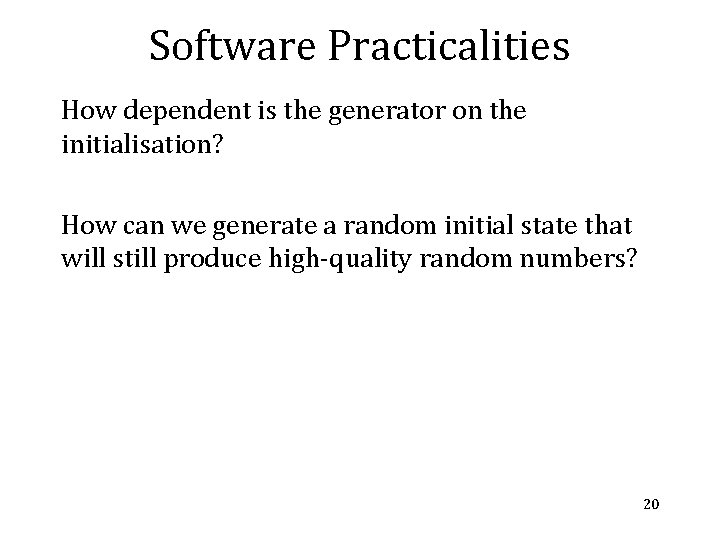Software Practicalities How dependent is the generator on the initialisation? How can we generate a random initial state that will still produce high-quality random numbers? 20Truly Random Numbers While most computers are incapable of producing truly random numbers there are some modern devices capable of generating a truly random number. 21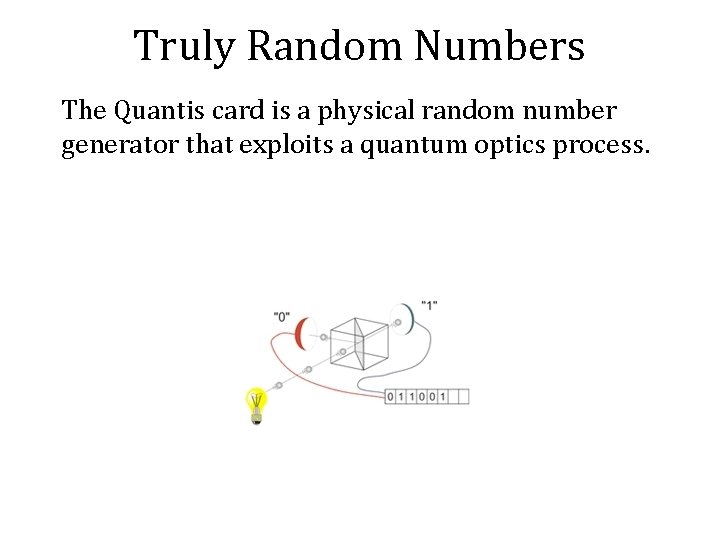Truly Random Numbers The Quantis card is a physical random number generator that exploits a quantum optics process.Truly Random Numbers A simulation that uses a truly random number is not repeatable. These quantum random number generators are not seeded and each simulation will be different. Any interesting occurrence or phenomena will not necessarily be reproducible.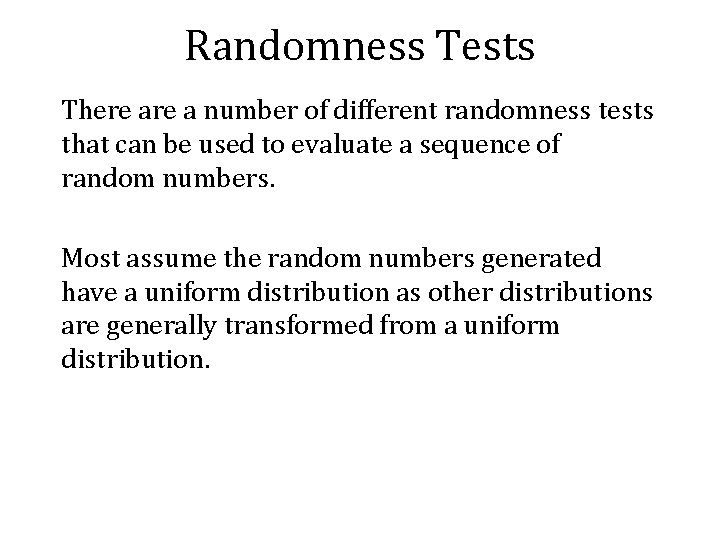Randomness Tests There a number of different randomness tests that can be used to evaluate a sequence of random numbers. Most assume the random numbers generated have a uniform distribution as other distributions are generally transformed from a uniform distribution.Randomness Tests One simple method to evaluate random number generators is a histogram test. Any sequence of uniform random numbers should produce an approximately flat histogram. The larger the number of random numbers tested, the flatter we expect the histogram to be.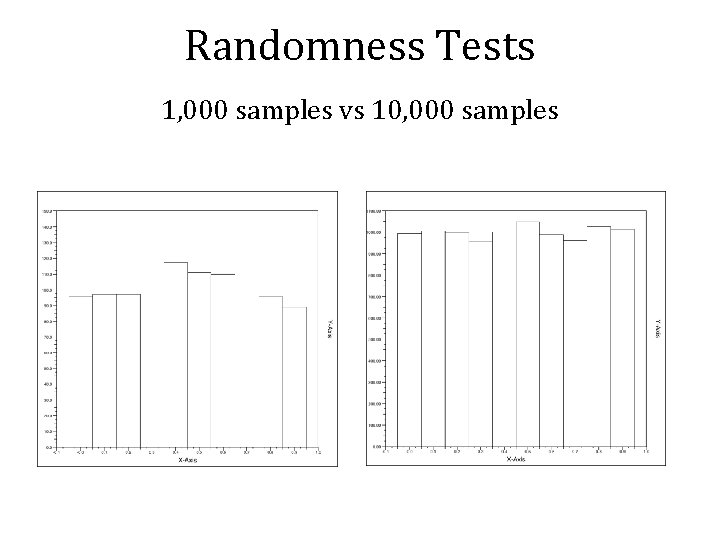Randomness Tests 1, 000 samples vs 10, 000 samples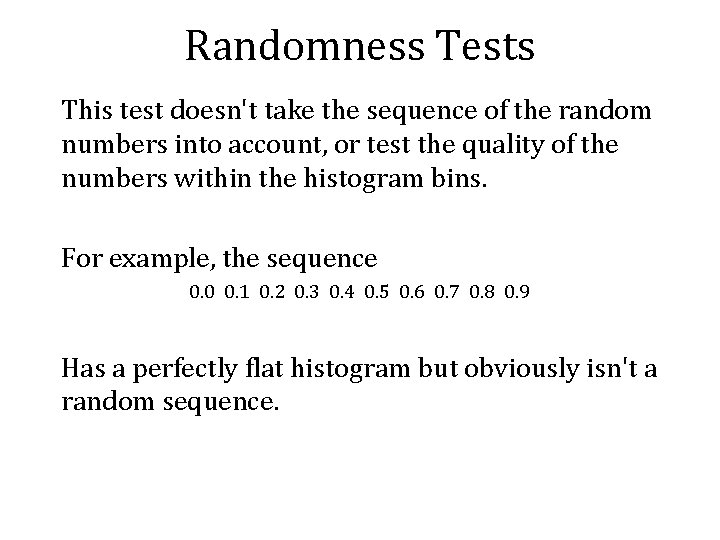Randomness Tests This test doesn't take the sequence of the random numbers into account, or test the quality of the numbers within the histogram bins. For example, the sequence 0. 0 0. 1 0. 2 0. 3 0. 4 0. 5 0. 6 0. 7 0. 8 0. 9 Has a perfectly flat histogram but obviously isn't a random sequence.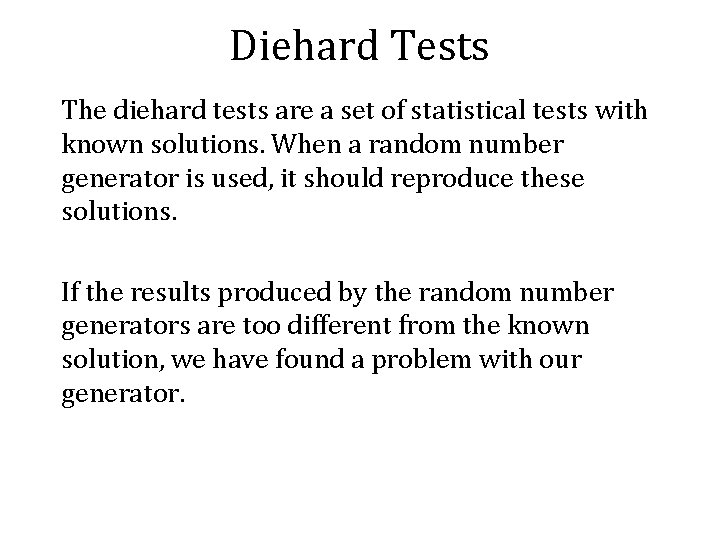Diehard Tests The diehard tests are a set of statistical tests with known solutions. When a random number generator is used, it should reproduce these solutions. If the results produced by the random number generators are too different from the known solution, we have found a problem with our generator.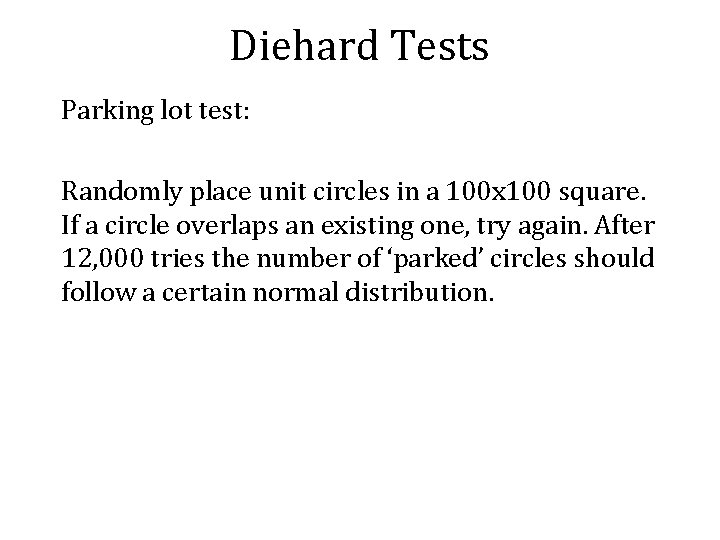Diehard Tests Parking lot test: Randomly place unit circles in a 100 x 100 square. If a circle overlaps an existing one, try again. After 12, 000 tries the number of ‘parked’ circles should follow a certain normal distribution.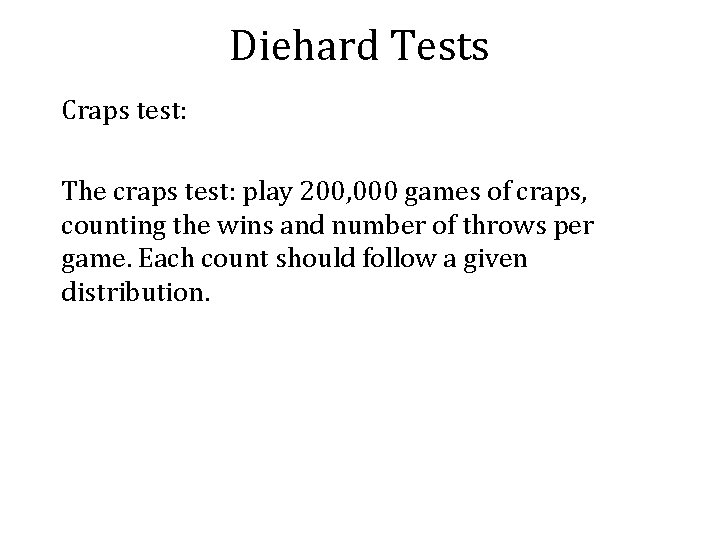Diehard Tests Craps test: The craps test: play 200, 000 games of craps, counting the wins and number of throws per game. Each count should follow a given distribution.Diehard Tests Overlapping permutations test: The Overlapping Permutations test: Analyze sequences of give consecutive random numbers. The 120 possible orderings should occur with statistically equal probability.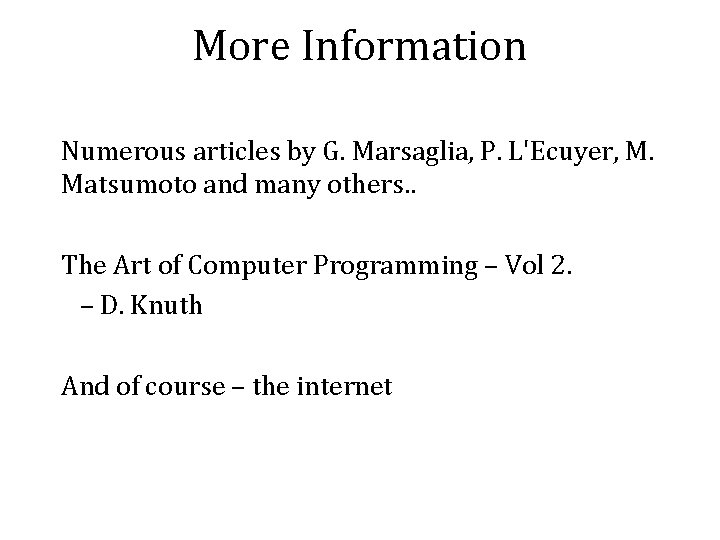More Information Numerous articles by G. Marsaglia, P. L'Ecuyer, M. Matsumoto and many others. . The Art of Computer Programming – Vol 2. – D. Knuth And of course – the internet